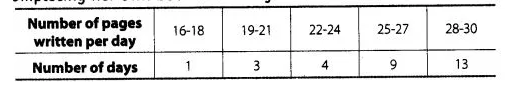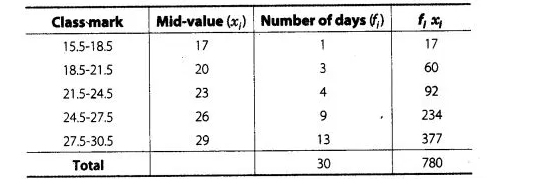# The following table gives the number of pages

Question:

The following table gives the number of pages written by Sarika for completing her own book for 30 days.Find the mean number of pages written per day.

Solution:

Since,Since, given data is not continuous, so we subtract 0.5 from the lower limit and add 0.5 in the upper limit of each class.

$\therefore$ $\operatorname{Mean}(\bar{x})=\frac{\Sigma f_{j} x_{i}}{\Sigma f_{i}}=\frac{780}{30}=26$

Hence, the mean of pages written per day is 26.